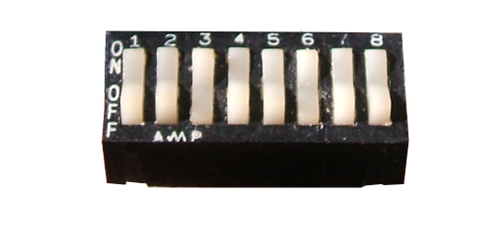# Unravelling CV29JOHN CHANDLER looks at what digital users can do with this useful command variable.

See more ELECTRICS

CV29 is probably one of the most useful, but also one of the most feared, CVs in a DCC locomotive decoder. It allows configuration of up to 8 parameters which contribute to the operation of the decoder and can bite if not set correctly.

Having an Engineering background with a focus on computers and control systems, I have used several number systems other than the 'standard' denary (base 10) system. CV29 is based on the binary system (base 2), probably the 'lowest' form of number system around. In short, it is the basis of digital computing; it has two states, on (usually represented as 1) or off (usually represented as 0). (There are 10 types of people in the world: those who understand binary and those who don't!)

As an aside, we’re all using different base numbers every day! Take, for example, time. 60 seconds per minute (= base 60), 60 minutes per hour (again, base 60) 24 hours per day (= base 24). Horse racing: 8 furlongs = 1 mile (= base 8). Nautically, there are 6 feet to the fathom (= base 6). Even on the railways where they still use miles and chains, 80 chains = 1 mile (= base 80) and, combining two of these, there are 10 chains to a furlong (hey, we’re back to good old base 10!). In binary, 1 + 1 = 10; 10 + 1 = 11; 11 + 1 = 100.

CV29 comprises effectively 8 switches which are either on or off; in computing terms, each one is called a bit. 8 bits can be used to represent any decimal number from 0 (all off) to 255 (all on).For illustrative purposes only - an 8 switch DIP switch, which does the same thing as CV29 but mechanically.

Let's have a look at what CV29 controls:

BitDefault SettingDecimal ValueFunction Controlled
001Changes the direction of travel. (0 = Normal, 1 = Opposite) Useful if there are decoders at each end of the train so that they act consistently.
112Default number of speed steps used. (0 = 28, 1 = 128)
214DC Operation (0 = DCC Operation, 1 = both DC and DCC Operation)
308Railcom (0 = Off, 1 = On)
4016Custom Speed Curve (0 = Standard Settings using CV2, CV6 and CV5, 1 = Custom Speed Curve as programmed in CVs 67 thru' 94)
5032Long Locomotive Address (0 = Short Address from CV1, 1 = Long Address from CVs 17 & 18)
6064Not commonly used. Refer decoder manufacturer's instructions
70128Not commonly used. Refer decoder manufacturer's instructions
Default:6

To calculate the value required, decide which bits need to be turned on then add the corresponding decimal values together and set CV29 to this value. In the above table, bits 1 (= 2) and 2 (= 4) are turned on; 2 + 4 = 6, the value shown as default in the table.